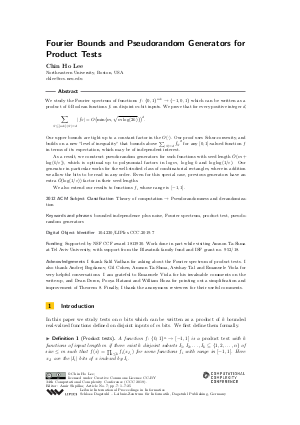Document# Fourier Bounds and Pseudorandom Generators for Product Tests

### Author Chin Ho Lee## File

LIPIcs.CCC.2019.7.pdf
• Filesize: 0.59 MB
• 25 pages

## Acknowledgements

I thank Salil Vadhan for asking about the Fourier spectrum of product tests. I also thank Andrej Bogdanov, Gil Cohen, Amnon Ta-Shma, Avishay Tal and Emanuele Viola for very helpful conversations. I am grateful to Emanuele Viola for his invaluable comments on the write-up, and Dean Doron, Pooya Hatami and William Hoza for pointing out a simplification and improvement of Theorem 8. Finally, I thank the anonymous reviewers for their useful comments.

## Cite As

Chin Ho Lee. Fourier Bounds and Pseudorandom Generators for Product Tests. In 34th Computational Complexity Conference (CCC 2019). Leibniz International Proceedings in Informatics (LIPIcs), Volume 137, pp. 7:1-7:25, Schloss Dagstuhl - Leibniz-Zentrum für Informatik (2019)
https://doi.org/10.4230/LIPIcs.CCC.2019.7

## Abstract

We study the Fourier spectrum of functions f : {0,1}^{mk} -> {-1,0,1} which can be written as a product of k Boolean functions f_i on disjoint m-bit inputs. We prove that for every positive integer d, sum_{S subseteq [mk]: |S|=d} |hat{f_S}| = O(min{m, sqrt{m log(2k)}})^d . Our upper bounds are tight up to a constant factor in the O(*). Our proof uses Schur-convexity, and builds on a new "level-d inequality" that bounds above sum_{|S|=d} hat{f_S}^2 for any [0,1]-valued function f in terms of its expectation, which may be of independent interest. As a result, we construct pseudorandom generators for such functions with seed length O~(m + log(k/epsilon)), which is optimal up to polynomial factors in log m, log log k and log log(1/epsilon). Our generator in particular works for the well-studied class of combinatorial rectangles, where in addition we allow the bits to be read in any order. Even for this special case, previous generators have an extra O~(log(1/epsilon)) factor in their seed lengths. We also extend our results to functions f_i whose range is [-1,1].

## Subject Classification

##### ACM Subject Classification
• Theory of computation → Pseudorandomness and derandomization
##### Keywords
• bounded independence plus noise
• Fourier spectrum
• product test
• pseudorandom generators

## Metrics

• Access Statistics
• Total Accesses (updated on a weekly basis)
0